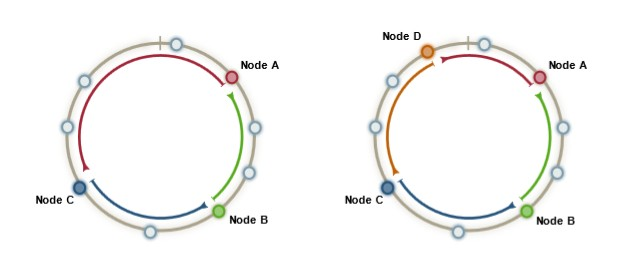# Cryptography - (Ciphertext|cyphertext|Message Digest|Digest)

In cryptography, ciphertext or cyphertext is the result of encryption performed on plaintext using an algorithm, called a cipher.

## Context

### Message digest

In the context of hashing / data integrity, the cyphertext is known as digest or message digest in the context of

The message is digested by a hash function

Discover MoreAnsible - Vault

A vault is the encrypted format of data through the combination of a label and a password known as vault id Encrypted data may be: a whole file or a property value This is available since Ansible...Crypto

graphy is the science of cryptographic algorithms (known as Cipher) that implements the following functionalities: Data Integrity - the message (data) was not altered during transit Authenticity -...Crypto - initialization vector (IV) or starting variable (SV)

Most block cipher modes require a unique binary sequence, often called an initialization vector (IV), for each encryption operation. same as salt ? An initialization vector (IV) is a data block that...Cryptography - Algorithm (called a Cipher)

A cipher is an algorithm that encrypt a plain text message into a ciphertext. Cipher algorithm can be categorized by the key that they used as parameters. No key symmetric one (using one key) ...Cryptography - Decryption (Deciphering)

A process from a cipher that transforms encrypted data into the original usable dataCryptography - Encryption (Enciphering)

Encryption is the function of a cipher that transforms usable data into an unreadable form. It provides confidentiality if a secret is used in the encryption. There is two class of encryption. ...Cryptography - Hash

A hash function is an encryption crypto algorithm that takes as data as input (possibly large and of variable-sized) and produces a short fixed-length integer value (generally printed as an hexadecimal...Cryptography - Input Data (plain text | clear text | message)

Plain text is a text that you want to send. Plain text is also known as Cleartext Usable text Message Even if its name contains text, the input can be any piece of data: file content, network...Cryptography - Key

A key is a parameter used in a cipher algorithm that determines the encryption operation (forward) and the decryption operation (backward). It's the only secret parameter that protect the anonymity...How to protect numerical Id ? (obfuscate, id encryption)

This page is how to obfuscate / protect a internal id. So that even if they are public, they cannot be guessed. This is mostly used to prevent guessing your data. For instance, you can estimate...